LLC DCT+BUCK/BOOST组成双向DCDC的控制
1天前
TCM模式的多相交错BUCK/BOOST控制实现与仿真
2天前
TCM模式BUCK/BOOST在数字环境中控制实现与思考
3天前

4天前

1星期前# 准比例谐振控制器(QPR)的离散化与代码实现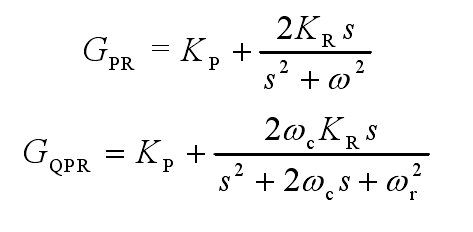QPR因为缓解PR在谐振点的增益以及有更好的适应性，而被广泛使用。传递函数中的wc用来调节QPR控制器在谐振点的增益带宽，wc越小则QPR在谐振的增益峰值越接近PR。kr是QPR控制器在谐振点的增益值，wr则是QPR控制器的设定谐振频率。参考文献1中展示了一张不同的kr和wc的QPR的bode图，可见：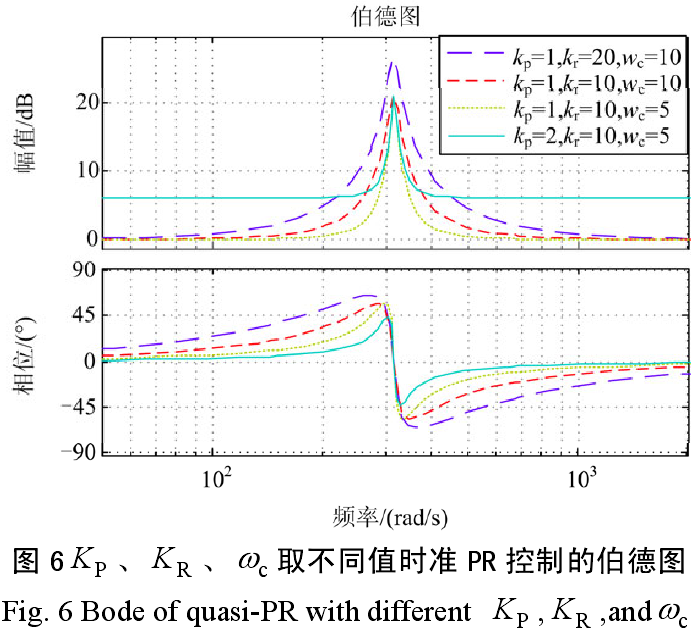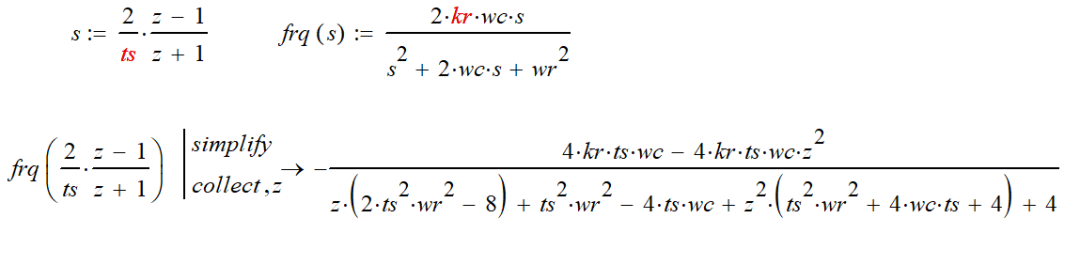ts_x_ts = ts * ts;

wr_x_wr = wr * wr;

div_x = ts * ts * wr * wr + 4.0 * wc * ts + 4.0;

coeff_B0 = (4.0 * kr * wc * ts)/div_x;

coeff_B1 = 0;

coeff_B2 = -1*coeff_B0;

coeff_A1 = (2.0 * ts_x_ts * wr_x_wr - 8.0)/div_x;

coeff_A2 = (ts_x_ts * wr_x_wr - 4.0 * ts * wc + 4)/div_x;

pr_1st_z = c2d(pr_1st, ts, 'tustin')  %Matlab自带函数

pr_1st_Z = tf([coeff_B0 coeff_B1 coeff_B2], [1 coeff_A1 coeff_A2], ts)  %自己手动

pr_1st_z =

0.03912 z^2 - 0.03912

----------------------

z^2 - 1.992 z + 0.9922

Sample time: 2.5e-05 seconds

Discrete-time transfer function.

pr_1st_Z =

0.03912 z^2 - 0.03912

----------------------

z^2 - 1.992 z + 0.9922

Sample time: 2.5e-05 seconds

Discrete-time transfer function.

w(1) = inputl(i)  - w(2) * a_coff(2) -  w(3) * a_coff(3);

yout(i) = (w(1) * b_coff(1) + w(2) * b_coff(2) +w(3) * b_coff(3));

w(3) = w(2);

w(2) = w(1);

lpf = (flpf*2*pi/(s + flpf*2*pi));

sys_pr = kp + lpf * (pr_1st + pr_3st + pr_5st + pr_7st + pr_9st + pr_11st);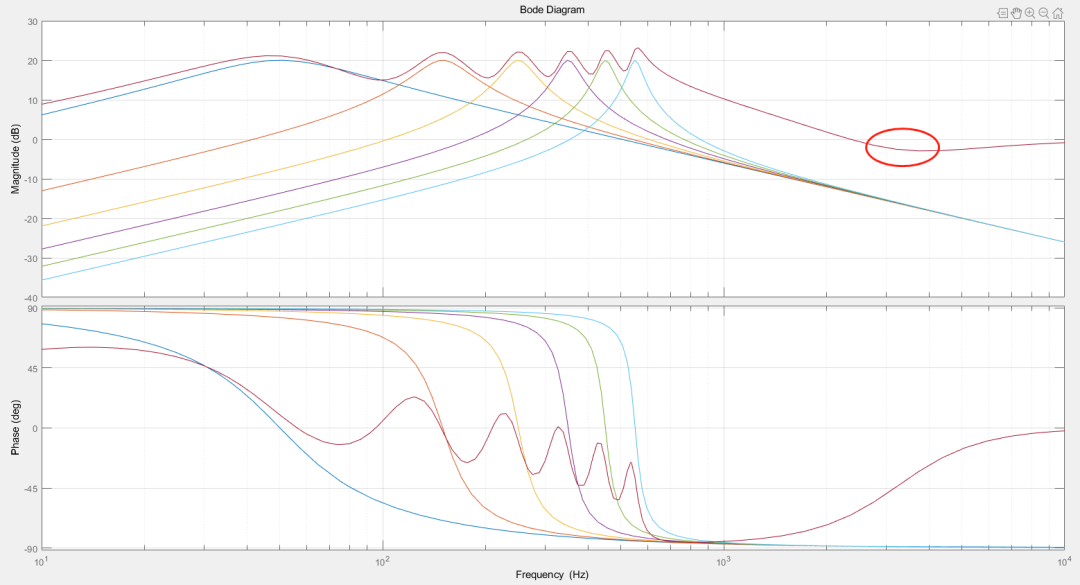（3KHZ后 LPF开始起到作用）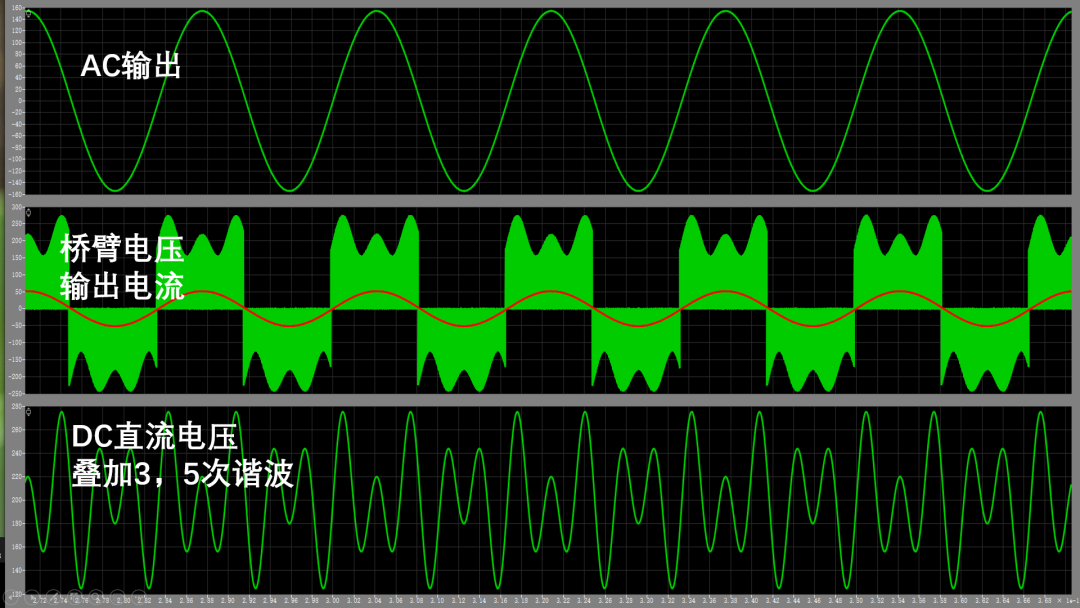1，基于准PQ调节器电流双闭环LCL三相并网逆变器控制  雷亚雄, 李建文, 李永刚 （华北电力大学新能源电力系统国家重点实验室，河北 保定 071003）

0/200